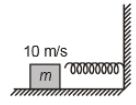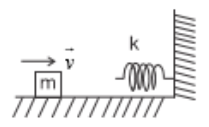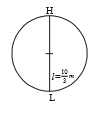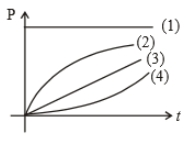A spring 40 mm long is stretched by the application of a force. If 10 N force required to stretch the spring through 1 mm, then work done in stretching the spring through 40 mm is

(a) 84J

(b) 68J

(c) 23J

(d) 8J

Concept Questions :-

Elastic potential energy
NEET 2021 - Achiever Batch - Aryan Raj Singh

Difficulty Level:

Two springs with spring constants ${k}_{1}$ = 1500 N/m and ${k}_{2}$ = 3000 N/m are stretched by the same force. The ratio of potential energy stored in the springs will be

1. 2:1

2. 1:2

3. 4:1

4. 1:4

Concept Questions :-

Elastic potential energy
NEET 2021 - Achiever Batch - Aryan Raj Singh

Difficulty Level:

A block of mass 2 kg moving with velocity of 10 m/s on a smooth surface hits a spring of force constant $80×{10}^{3}$ N/m as shown. The maximum compression in the spring is1. 5 cm

2. 10 cm

3. 15 cm

4. 20 cm

Concept Questions :-

Elastic potential energy
NEET 2021 - Achiever Batch - Aryan Raj Singh

Difficulty Level:

A body starts moving from rest in straight line under a constant power source. Its displacement in time t is proportional to

(1) ${t}^{1/2}$

(2) t

(3) ${t}^{3/2}$

(4) ${t}^{2}$

Concept Questions :-

Power
NEET 2021 - Achiever Batch - Aryan Raj Singh

Difficulty Level:

A block of mass m = 25 kg on a smooth horizontal surface with a velocity $\stackrel{⇀}{v}$ = 3$m{s}^{-1}$ meets the spring of spring constant k = 100 N/m fixed at one end as shown in the figure. The maximum compression of the spring and velocity of the block as it returns to the original position respectively are1.  1.5m, -3$m{s}^{-1}$

2.  1.5m, 0$m{s}^{-1}$

3.  1.0m, 3$m{s}^{-1}$

4.  0.5m, 2$m{s}^{-1}$

Concept Questions :-

Elastic potential energy
NEET 2021 - Achiever Batch - Aryan Raj Singh

Difficulty Level:

The velocity, given to the block of mass (m), is to rotate it in a circle of radius l. Calculate the height (h) where the block leaves the circle.

(1) $\frac{3l}{2}$

(2) $\frac{4l}{3}$

(3) $\frac{5l}{4}$

(4) None of these

Concept Questions :-

Work-Energy theorem
NEET 2021 - Achiever Batch - Aryan Raj Singh

Difficulty Level:If length of string is

1.  10 m/s

2.  20 m/s

3.  $\frac{10}{\sqrt{2}}\mathrm{m}/\mathrm{s}$

4.

Concept Questions :-

Work-Energy theorem
NEET 2021 - Achiever Batch - Aryan Raj Singh

Difficulty Level:

The relation between velocity (v) and time (t) is $v\propto \sqrt{\mathrm{t}}$, then which one of the following quantity is constant?

1.  Force

2.  Power

3.  Momentum

4.  Kinetic Energy

Concept Questions :-

Power
NEET 2021 - Achiever Batch - Aryan Raj Singh

Difficulty Level:

A particle is moving on the circular path of the radius (R) with centripetal acceleration ${a}_{c}={k}^{2}R{t}^{2}$. Then the correct relation showing power (P) delivered by net force versus time (t) is1. 1

2. 2

3. 3

4. 4

Concept Questions :-

Power
NEET 2021 - Achiever Batch - Aryan Raj Singh

Difficulty Level:

A steel wire can withstand a load up to 2940 N. A load of 150 kg is suspended from a rigid support. The maximum angle through which the wire can be displaced from the mean position, so that the wire does not break when the load passes through the position of equilibrium, is (2008 E)

(1) 30$°$

(2) 60$°$

(3) 80$°$

(4) 85$°$

Concept Questions :-

Work-Energy theorem